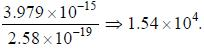Courses

# Adsorption, Surface Chemistry

## 20 Questions MCQ Test GATE Chemistry Mock Test Series | Adsorption, Surface Chemistry

Description
This mock test of Adsorption, Surface Chemistry for Chemistry helps you for every Chemistry entrance exam. This contains 20 Multiple Choice Questions for Chemistry Adsorption, Surface Chemistry (mcq) to study with solutions a complete question bank. The solved questions answers in this Adsorption, Surface Chemistry quiz give you a good mix of easy questions and tough questions. Chemistry students definitely take this Adsorption, Surface Chemistry exercise for a better result in the exam. You can find other Adsorption, Surface Chemistry extra questions, long questions & short questions for Chemistry on EduRev as well by searching above.
QUESTION: 1

### Calculate how long a hydrogen atom will remains on the surface of a solid at 1000 K if its desorption activation energy is 15 kJ mol and assume that

Solution:

According to desorption activation energy: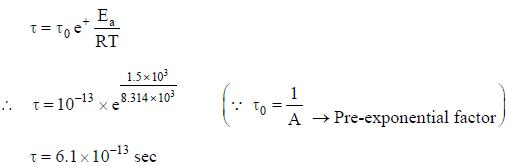QUESTION: 2

### The volume of gases NH3, CO2 and H2 gas adsorbed by 1 gm charcoal at 300 K lie in the order ?

Solution:

More readily soluble and easily liquifiable gases such as NH3/HC1/C12 and SO2, are adsorbed more than H2/N2.
i.e.. adsorption
proportional to intemiolecular interaction,
proportional to molecular mass,
proportional to ease of liquidation,
proportional to 'a’.
proportional to ciitical temperature (Te.
Therefore, order of adsoiption of gases:
SO2 > NH3 > HC1 > CO2, > CH4 > CO > N2 > H2

*Answer can only contain numeric values
QUESTION: 3

### The adsorption of a gas an a solid surface was found to follow a langmuir isotherm with K = 4 Pa-1 at a temp of 25 °C. The pressure of a gas required to achieve a fractional surface coverage of 90 % is _____________________________ (in Pa), (upto two decimal places)

Solution: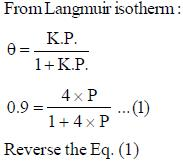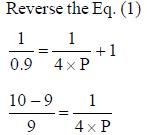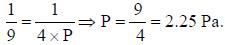QUESTION: 4

Which of the following factor's influencing the adsorption?

Solution:
QUESTION: 5

Following plot represents the varition of temperature T with pressure for a definite extent of adsorption (xin) is called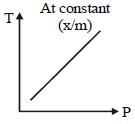Solution:
QUESTION: 6

Which among the following statements are NOT collects w.r.t. adsorption of gases on a solid ?

Solution:
QUESTION: 7

Graph between log (x in) and log (P) is straight line at angle of 45° with intercept is 0.602 ?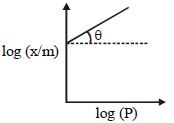The extent of adsorption (x in) at a pressure of 1 atm is

Solution:

According to Freundlich adsorption isotherm: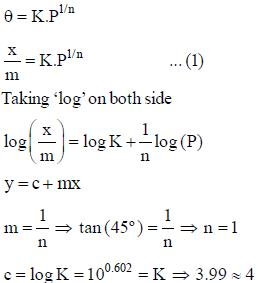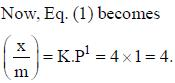QUESTION: 8

Which of the following animation 's are correct in which B .E .T equation reduced into Langmuir ?

Solution:

B.E.T equation: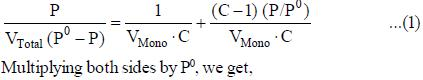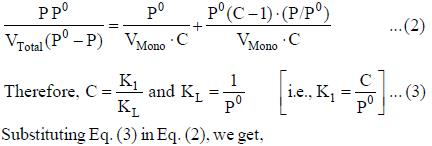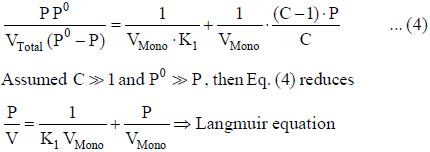QUESTION: 9

Which of the inherent assumption in the Langmuir model of surface adsorption are ?

Solution:
QUESTION: 10

Following is the varition of physical as adsoiption with temperature ?

Solution:
QUESTION: 11

Which of the following plot does not corresponds to the adsorption isotherm ?

Solution:

Because:
(a) Amount of adsorption keeps on rising in each case with increase in pressure
E .g: Adsorptio n of Br2, on silica get at 80°C
(b) Multilayer physical adsorption on non-porous solid.
E.g: Adsorption of N, on silica gel.
(c) Langmuir type isotherm.
E.g: H2 and N2 adsorbed on charcoal at 180 °C.

QUESTION: 12

The adsorption of a gas is described by the L angmuir adsorption isotherm with K = 0.3 kPa-1. A t a pressure of 0.2 kPa. the if actional coverage is

Solution: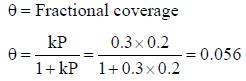QUESTION: 13

Which of the following expression are CORRECT ?

Solution:
*Answer can only contain numeric values
QUESTION: 14

At 273 K, N2 is absorbed on a niica surface. A plot of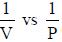( V in m3 and P in torr) gives a straight line with a slope equal to 2x 10-5 torr m-3 and intercept equivalent to Vm is equal to 4 x 10-8 m3. The ad solution coefficient is _____________________ x 1012 (torr-1) ( upto two decimal places)

Solution:

As we know that.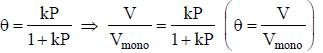Reverse the reactioin,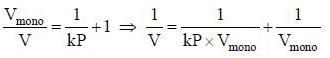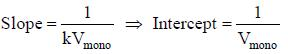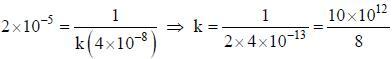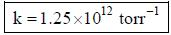*Answer can only contain numeric values
QUESTION: 15

At 273 K, H2 is absorbed on a mica surface. A plot of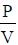vs P (V in m3 and P in Pa) gives a straight line with a slope equal to 2 cm-3 and intercept is 3x10-3 cm-3 Pa respectively. The adsorption coefficient is ____________________ x 103 Pa. (upto two decimal places)

Solution:

From Langmuir isotherm.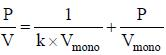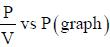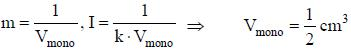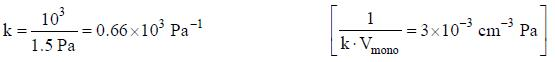*Answer can only contain numeric values
QUESTION: 16

Calculate the adsorption of a dye on activated carbon at 25°C, where k = 0.025, n = 0.5 and C = 0.04.
Based on the Freundlich isotherm.

Solution:

Given data
n = 0.5
Kd = 0.025
C = 0.04
Substitute the values in the corresponding equation
q = Kd C(1/n)
q = (0.025) (0.04)(1/0.5)
q = 0.040.

QUESTION: 17

The time for which the oxygen atom remains adsorbed on a tungsten surface is 0.36 at 2550 K and 3.49 at 2360 K. Calculate the activation energy of desorption of oxygen atom.

Solution:

From integrated Arrhenius type equation: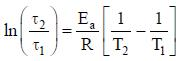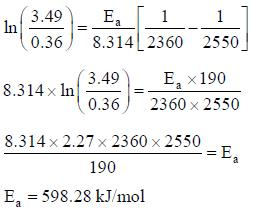QUESTION: 18

Ail organic fatty acid forms a surface film on water that obeys the two-Dimensional ideal gas law. If the surface tension lowering is 10 mNm-1 at 25°C. The surface area per adsorbed molecules is

Solution:

Surface film pressure equation (π) = τ2RT
Surface excess concentration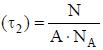Therefore, π = surface tension. N = number of molecules.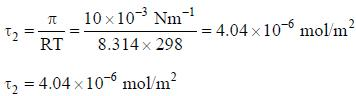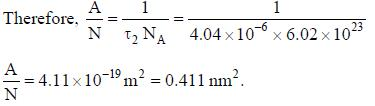QUESTION: 19

An 18°C . the surface tension (γ) of an aqueous solution of butyric acid is represented by the equation γ = γ0 -29 .8 log( 19.64 c2 + 1 ) where γ0 is surface tension of water is 0.0073 Nm-1 and cis the bulk concentration of the solute. Using the Gibbs adsorption isotherm to estimate the surface excess of butyric acid at c2 is equal to 0.01 mol dm-3

Solution: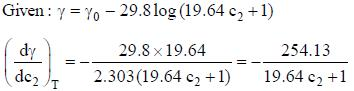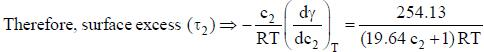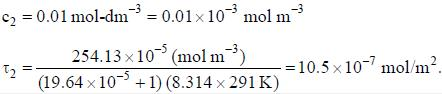*Answer can only contain numeric values
QUESTION: 20

The number of molecules of CETANOL absorbate of cross-section area 2.58 x 10-19 m2 can be observed on the surface of a spherical drop of dodecane of radius 17 .8 pm is ________________ x 104. (Round off to two decimal places)

Solution:

Area = 2.58 x 10-19m2 (occupied by single molecules)
1= 17.8 nm= 17.8 x 10-9 m
Total surface area of docedane = 4 πr2
= 4 x 3.14 x (17.8 x 10-9)2
= 3.979 x 10-15 m2
Therefore, total surface area = Area occupied by single molecules x Number of molecules
3.979 x 10-15 = 2.58x 10-19 x Number of molecules
Number of molecules =